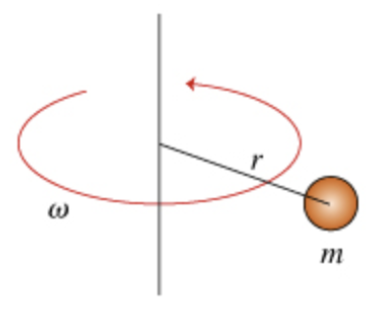# Problem: Consider a particle of mass m that is revolving with angular speed ω around an axis. The perpendicular distance from the particle to the axis is r. (Figure 1) 1.) Find the kinetic energy K of the rotating particle. Express your answer in terms of m, r, and ω.2.) Find the moment of inertia Ihoop of a hoop of radius r and mass m with respect to an axis perpendicular to the hoop and passing through its center. Express your answer in terms of m and r.

###### FREE Expert Solution

Kinetic energy:

$\overline{){\mathbf{K}}{\mathbf{=}}\frac{\mathbf{1}}{\mathbf{2}}{\mathbf{m}}{{\mathbf{v}}}^{{\mathbf{2}}}}$

1.

From the equation, we get v as:

v = rω

92% (307 ratings)###### Problem Details

Consider a particle of mass m that is revolving with angular speed ω around an axis. The perpendicular distance from the particle to the axis is r. (Figure 1)1.) Find the kinetic energy K of the rotating particle. Express your answer in terms of m, r, and ω.

2.) Find the moment of inertia Ihoop of a hoop of radius r and mass m with respect to an axis perpendicular to the hoop and passing through its center. Express your answer in terms of m and r.